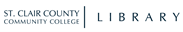Library Home PageHomeSearchMy AccountBasicAdvancedHistorySearch: WORD(s) anywhereTITLE begins with ...TITLE word(s)AUTHOR (last name first)AUTHOR word(s)SUBJECT begins with ...SUBJECT word(s)VIDEO TITLE word(s)Bib No. Refine Search > You're searching: St Clair County Community College
Item InformationHoldings Choice Review Summary More Content More by this author Hutchinson, I. H. (Ian H.), 1951- Subjects Numerical analysis. Mensuration. Number concept. Matrices. Mathematics -- Study and teaching. Browse Catalog by author: Hutchinson, I. H. (Ian H.), 1951- by title: A student's guide to... MARC Display
A student's guide to numerical methods / Ian H. Hutchinson, Massachusetts Institute of Technology.
by Hutchinson, I. H. (Ian H.), 1951-Subjects
• Numerical analysis.
•
• Mensuration.
•
• Number concept.
•
• Matrices.
•
• Mathematics -- Study and teaching.
• ISBN:
 9781107095670 1107095670 9781107479500 1107479509
Description:
 xiv, 207 pages : illustrations ; 24 cm
Contents:
 pt. I. Fitting functions to data. Exact fitting ; Approximate fitting ; Tomographic image reconstruction ; Efficiency and non-linearity -- pt. II. Ordinary differential equations. Reduction to first order ; Numerical integration of initial-value problems ; Multidimensional stiff equations : implicit schemes ; Leap-frog schemes -- pt. III. Two-point boundary conditions. Examples of two-point problems ; Shooting ; Direct solution ; Conservative differences, finite volumes -- pt. IV. Partial differential equations. Examples of partial differential equations ; Classification of partial differential equations ; Finite-difference partial derivatives -- pt. V. Diffusion. parabolic partial differential equations. Diffusion equations ; Time-advance choices and stability ; Implicit advancing matrix method ; Multiple space dimensions ; Estimating computational cost -- pt. VI. Elliptic problems and iterative matrix solution. Elliptic equations and matrix inversion ; Convergence rate ; Successive over-relaxation ; Iteration and non-linear equations -- pt. VII. Fluid dynamics and hyperbolic equations. The fluid momentum equation ; Hyperbolic equations ; Finite differences and stability -- pt. VIII. Boltzmann's equation and its solution. The distribution function ; Conservation of particles in phase-space ; Solving the hyperbolic Boltzmann equation ; Collision term -- pt. IX. Energy-resolved diffusive transport. Collisions of neutrons ; Reduction to multigroup diffusion equations ; Numerical representation of multigroup equations -- pt. X. Atomistic and particle-in-cell simulation. Atomistic simulation ; Particle-in-cell codes -- pt. XI. Monte Carlo techniques. Probability and statistics ; Computational random selection ; Flux integration and injection choice -- pt. XII. Monte Carlo radiation transport. Transport and collisions ; Tracking, tallying, and statistical uncertainty -- pt. XIII. Next steps. Finite-element methods ; Discrete Fourier analysis and spectral methods ; Sparse-matrix iterative Krylov solution ; Fluid evolution schemes -- Appendix A. Summary of matrix algebra. Vector and matrix multiplication ; Determinants ; Inverses ; Eigenanalysis.Copy/Holding information
 Call No. Collection Status Location QA39.3 .H92 2015 Circulating Books Checked In St. Clair County Community College Add Copy to MyList

 Format: HTMLPlain textDelimited Subject: Email to:

 Horizon Information Portal 3.24_8266© 2001-2013 SirsiDynix All rights reserved.
SC4 Library Catalog Interface